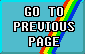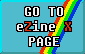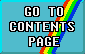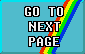# FIRST STEPS IN MACHINE CODE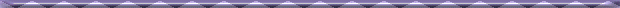## PART 4: BITS AND STACKS#### An introduction to Z80 Machine Code by David Nowotnik.

Ever wondered how a computer remembers where to turn to after completing a subroutine? All will be revealed in this, the fourth part of my machine code series. And, we will see lots of ways in which the smallest unit of memory, the bit, can be utilised to produce interesting machine code routines. But first, the stack!#### THE STACK

So far, we've dealt with many instructions to transfer bytes from one place to another - mainly from one register to another, and between memory and the registers. There is a special store of bytes of information in RAM called 'the stack', which has its own set of machine code instructions. The curious thing about the stack is that you usually don't have to worry about where in RAM it is stored; the processor does this for you automatically. You can change the position of the stack if you want to (with special machine code instructions), but it is usual to leave it where the processor puts it after power-up, which is just below RAMTOP.

What is 'the stack'? It's a 'pile' of bytes starting, as I said, just below RAMTOP, and building in a downward direction in RAM. The purpose of the stack is to have a temporary store of information (bytes) which you can dump there with a simple one byte instruction, then remove just as simply. It works something like this:

There is a special two byte register in the Z80 called SP, the stack pointer, which contains the first vacant address in the stack. If you want to store some information temporarily then you use the instruction PUSH to place that information (bytes) onto the stack. You can only PUSH onto the stack the value in a pair of registers; for example, PUSH HL, PUSH DE, PUSH BC, and PUSH AF (the 'A' register and the flag register combined) are the only PUSH instructions permitted. When you PUSH values onto the stack, the SP register is decremented by two, so that it contains the new address of the next free position in the stack. The stack is built up by moving DOWN in memory.

To remove a value from the stack, the instruction POP is used, and the value 'POPed' from the stack can be placed in one of the register pairs. As part of the POP instruction, the SP register is twice incremented, to show once more the address of the next free place on the stack. All the opcodes for the PUSH and POP instructions are shown in the PUSH/POP table. All are one byte instructions.

##### VIEW PUSH/POP TABLE

The stack is designed to make life easier for programmers. For example, if you want to preserve the value in one of the registers while you use it for something else, simply PUSH the value onto the stack, then POP it off when you need it. There will be examples of this later on. To transfer the values from one register pair to another all you have to do is to PUSH one register pair value into the stack, then POP it into the other register pair. I demonstrated this in Part 3, although I didn't explain how it worked. The machine code routine in the demonstration of flags program (in Part 3) had as consecutive instructions PUSH AF, POP DE. This placed the values A and F onto the stack, then placed them in D and E, respectively. This enabled the F register to be copied into the E register, from where it can be more closely examined.

You have to be quite careful how you use the stack. It operates by a last-on, first-off principle, so you must get the order on and off just right. The stack is also used by the Z80 processor outside of your control. Here's the answer to the subroutine question!

When you call a subroutine, either in BASIC, or a machine code subroutine, the return address is dumped onto the stack. If you call several subroutines, the return addresses are placed onto the stack in the correct order, and you'll always return to the right place in the program after each RETURN instruction because of the last on, first off principle of the stack. It is important therefore, to make sure that the number of POP instructions within a subroutine balances the number of PUSH instructions (and not to POP a value off the stack before one is PUSHed there), otherwise you could end up removing a return address, and causing yourself some real headaches.#### WORKING WITH BITS

There are far more machine code instructions that deal with bits than there are ones which manipulate bytes. So, it wont be of surprise to you that I wont be covering all of them this time, and I'll be saving some for the next part of this series. However, having said that there is a very large number of instructions which can all be grouped into a relatively small number of categories. But before we start to examine some of these, it might be useful to re-examine what a bit just happens to be.

Every byte of memory or register in the Z80 processors is made up of 8 bits. Each bit is, effectively, an electrical switch; it has two states, on or off, which can be represented by the values 1 or 0. There are 256 possible combinations of ones and zeros in the eight bits that make up a byte, hence the value range that a byte can hold is 0 to 255.

The binary demonstration program shows how the values of bits are combined to make up the value of a byte. When you RUN the program, the first thing you have to do is enter a decimal value. The binary representation of that value (i.e. the way it is held as ones and zeros in a byte) is shown on the screen. These bits are numbered 0 to 7 from right to left. Then watch the screen while you get a display of how to calculate the decimal value of a byte. Every time there is a 1 in a bit, then the value of that bit is added to the total. See if you can work out the relationship between a bit value and its number (0 to 7). If you want to slow down the display, then increase the size of the loop in line 1000.

With the knowledge that each bit has the effective value of one or zero, it may not come as a surprise to learn that the simplest of machine code bit operations involves setting the value of a specified bit to 1, or 0, or testing whether a bits value is either 1 or 0. You can SET (bit = 1) or RESET (bit = 0) values, or test them with the BIT instruction, for any bit in the main registers, A, B, C, D, E, H, L, or a bit in a byte of memory addressed by HL. If you work out all the combinations, then there are 64 SET instructions, 64 for RESET, and 64 for BIT. All are shown in the SET/RESET/BIT table. The SET, RESET and BIT instructions are all two bytes long, and all have as the first byte of the instruction, the hex value CB.

##### VIEW SET/RESET/BIT TABLE

All the assembly language instructions for BIT, SET, and RESET require two arguments to complete the instruction; the first is the identifying number of the bit (0 to 7), and the second is the register, or byte (with (HL)) identifier. For example, SET 5, E places the value 1 in bit 5 of the E register, and RESET 1, L places the value 0 in bit 1 of the L register.

The BIT instruction tests the value of the specified bit, and places the result in the zero flag. If the bit value is 1, then 1 is placed in the Z flag. The Z flag can then be tested, as described in Part Three. For example, BIT 3, (HL) tests the value of bit 3 in a byte of memory identified by the value in HL.

Another group of machine code instructions which work on bits have the opcodes AND, OR, and XOR. The first two will be familiar to BASIC programmers; the machine code and BASIC instructions of AND and OR are related, although that may be difficult to understand while I explain how they work!

AND, OR, and XOR carry out bit-by-bit comparisons of two bytes. One byte must be in the A register, the other byte must exist in any register (including A), or a byte of memory addressed by HL, or a byte of data stored immediately after the operand in the machine code routine. The result of this comparison is stored in the A register, replacing the previous value there. The result will effect the values of the sign, zero, and parity flags. Therefore, AND, OR and XOR are very similar to arithmetic machine code operations, except that their function is described as logic rather than arithmetical.

##### VIEW EXAMPLES OF LOGICAL OPERATIONS PLUS TEST

The truth tables show the result of comparing two bits by one of the logic operations, AND, OR, or XOR. For example, if you AND a bit value 1 with a bit of value 0, then the result is 0. OR the same two values, and the result will be 1; XORing 1 and 0 also give you 1. In fact, OR and XOR are very similar, except that 1 OR 1 = 1, whereas 1 XOR 1 = 0.

How these truth tables work in practice is also shown above. Work your way through the three logic sums comparing each pair of bits with the corresponding truth table. Then try the three sums which do not have an answer. The answer does appear at the end of this article, together with the decimal equivalent of the binary answer.

You should be able to work out for yourself the decimal value - if you've practised using the binary demonstration program. You may well be asking by now "what is the use of these logical operators?" There will be some examples of them later on, but, in summary:

 AND Is used mainly for masking bits within a byte. Say you wanted to reset bits 0 to 3 (i.e. make them all 0). You could use the RESET command four times, but it is far easier to use the machine code command AND 11110000. As bits 0 to 3 in the operand are all zero, then (from the truth tables) the bits 0 to 3 in the result must also be zero. OR In a similar manner to AND, the instruction OR will set a block of bits so that they all have the value l. For example, OR 00001111 ensures that bits 0 to 3 will all be 1 in the result. XOR Allows comparisons of bits such that, if they are the same, then the result is 0, and if they are different, the resultant bit value is 1. When you PRINT OVER 1 on the Spectrum, you are, in fact using the XOR instruction. Think of a pixel in the INK colour as 1 and a pixel in the PAPER colour as 0 (which they are), and try a few PRINT OVER 1s to see the effect. AND, OR, and XOR opcodes appear in the AND/OR/XOR.
##### VIEW AND/OR/XOR TABLE#### EXAMPLES

The two examples use the same decimal loader as used in Part Three. The CLEAR instruction lowers RAMTOP and moves the stack to below the new RAMTOP, so giving you an area of RAM which is quite safe to use. Type out the loader, then add the DATA lines appropriate to the routine you want to try.

The first routine scans the display file, and inverts every bit (i.e. changes 1 to 0, and 0 to 1), so reversing INK and PAPER. The important instruction for this is XOR 255. The second routine scans the attribute file and toggles the flash bit (that is, turns FLASH on if it finds the bit off, and vice versa). So, from a non-flashing screen, you should get the entire screen flashing. Try it!

##### VIEW ASSEMBLY LANGUAGE LISTING - EXAMPLE ONE

Both examples display one way of overcoming a major error in the Z80. Surprisingly, when using DEC on a two byte register, it doesn't reset the zero flag when the double register holds a value of zero. So, when using, say, BC as a counter, JRNZ after DEC BC will not work when BC becomes zero. Its a common mistake amongst beginners to machine code to assume (naturally) that it does. The way to overcome this is shown in the examples. Having carried out the DEC BC, the values in B and C are (effectively) ORed (after the value in B is transferred to the A register). Only when both B and C contain zero will the result of the OR operation be zero. The zero flag is reset when the result of a logical operation is zero, so the flag can be tested after the OR operation.

##### VIEW ASSEMBLY LANGUAGE LISTINGS - EXAMPLE TWO

The final items for this part are the results of the tests. These are as follows:

AND: 00010001 (17);
OR: 11101110 (238);
XOR: 10100110 (166).

If you don't agree with these results, then try again, working through the program in the binary demonstration program and the examples in the truth tables.#### FromZX Computing Volume 2 Number 7June/July 1985